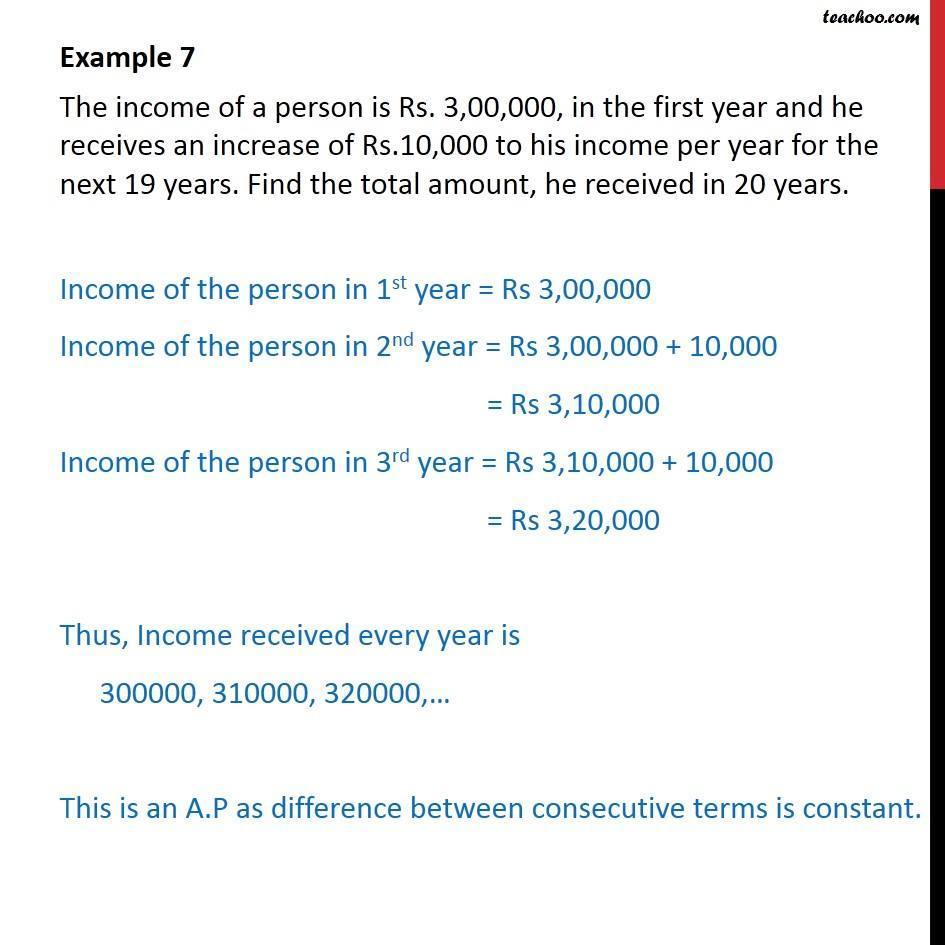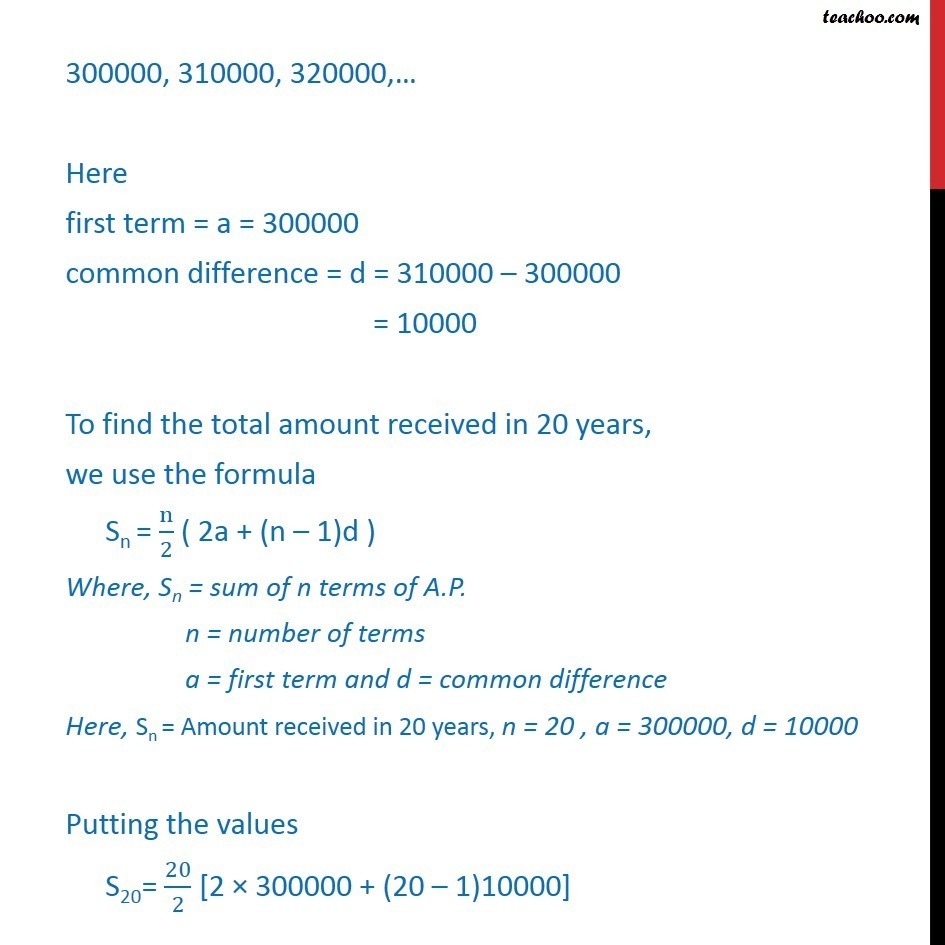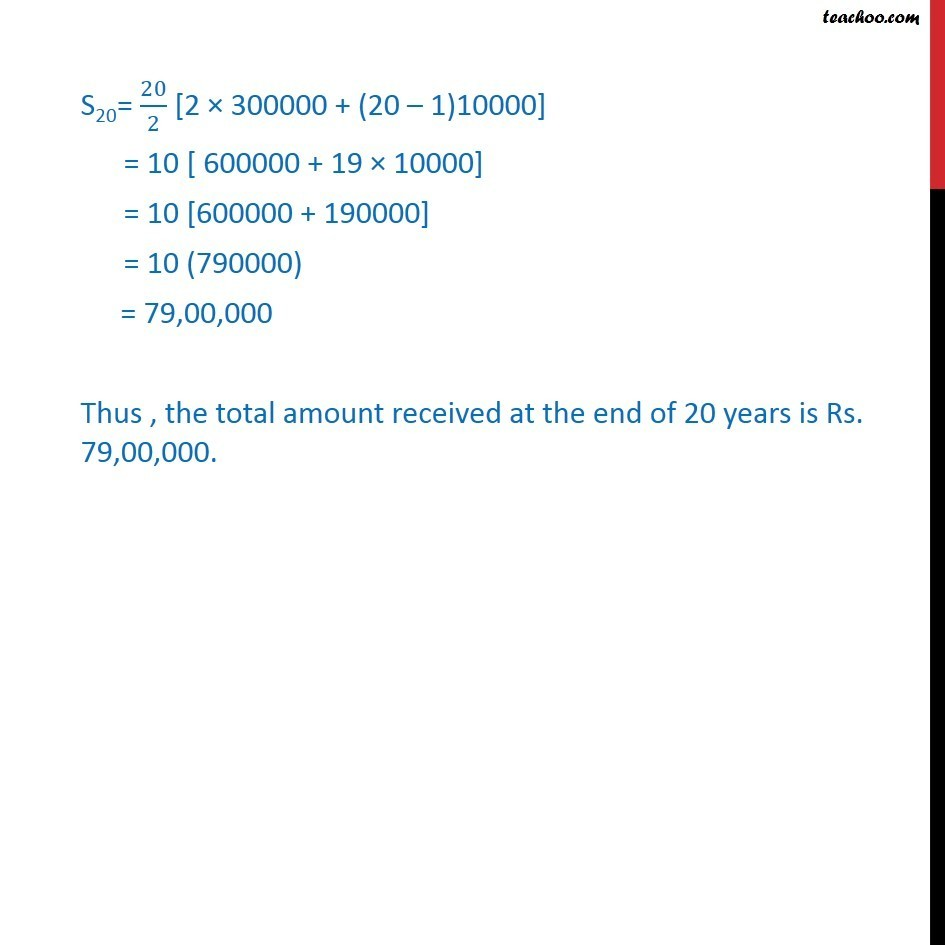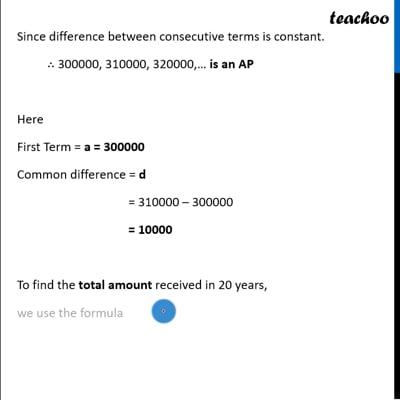Examples

Chapter 9 Class 11 Sequences and Series
Serial order wiseThis video is only available for Teachoo black users

Solve all your doubts with Teachoo Black (new monthly pack available now!)

### Transcript

Example 7 The income of a person is Rs. 3,00,000, in the first year and he receives an increase of Rs.10,000 to his income per year for the next 19 years. Find the total amount, he received in 20 years. Income of the person in 1st year = Rs 3,00,000 Income of the person in 2nd year = Rs 3,00,000 + 10,000 = Rs 3,10,000 Income of the person in 3rd year = Rs 3,10,000 + 10,000 = Rs 3,20,000 Thus, Income received every year is 300000, 310000, 320000, This is an A.P as difference between consecutive terms is constant. 300000, 310000, 320000, Here first term = a = 300000 common difference = d = 310000 300000 = 10000 To find the total amount received in 20 years, we use the formula Sn = n/2 ( 2a + (n 1)d ) Where, Sn = sum of n terms of A.P. n = number of terms a = first term and d = common difference Here, Sn = Amount received in 20 years, n = 20 , a = 300000, d = 10000 Putting the values S20= 20/2 [2 300000 + (20 1)10000] S20= 20/2 [2 300000 + (20 1)10000] = 10 [ 600000 + 19 10000] = 10 [600000 + 190000] = 10 (790000) = 79,00,000 Thus , the total amount received at the end of 20 years is Rs. 79,00,000.